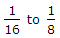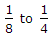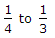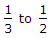# Mechanical Engineering - Hydraulics and Fluid Mechanics

### Exercise :: Hydraulics and Fluid Mechanics - Section 7

31.

The pressure at a stagnation point is always low.

 A. Agree B. Disagree

Answer: Option B

Explanation:

No answer description available for this question. Let us discuss.

32.

When an internal mouthpiece is running full, the discharge through the mouthpiece is twice the discharge when it is running free.

 A. Correct B. Incorrect

Answer: Option B

Explanation:

No answer description available for this question. Let us discuss.

33.

In two dimensional flow, the flow does not take place in a curve.

 A. True B. False

Answer: Option B

Explanation:

No answer description available for this question. Let us discuss.

34.

In order to avoid tendency of separation at throat in a venturimeter, the ratio of the diameter at throat to the diameter of pipe should be

 A.B.C.D.Answer: Option D

Explanation:

No answer description available for this question. Let us discuss.

35.

The ratio of the inertia force to the __________ is called Euler's number.

 A. pressure force B. elastic force C. surface tension force D. viscous force

Answer: Option A

Explanation:

No answer description available for this question. Let us discuss.

#### Current Affairs 2021

Interview Questions and Answers